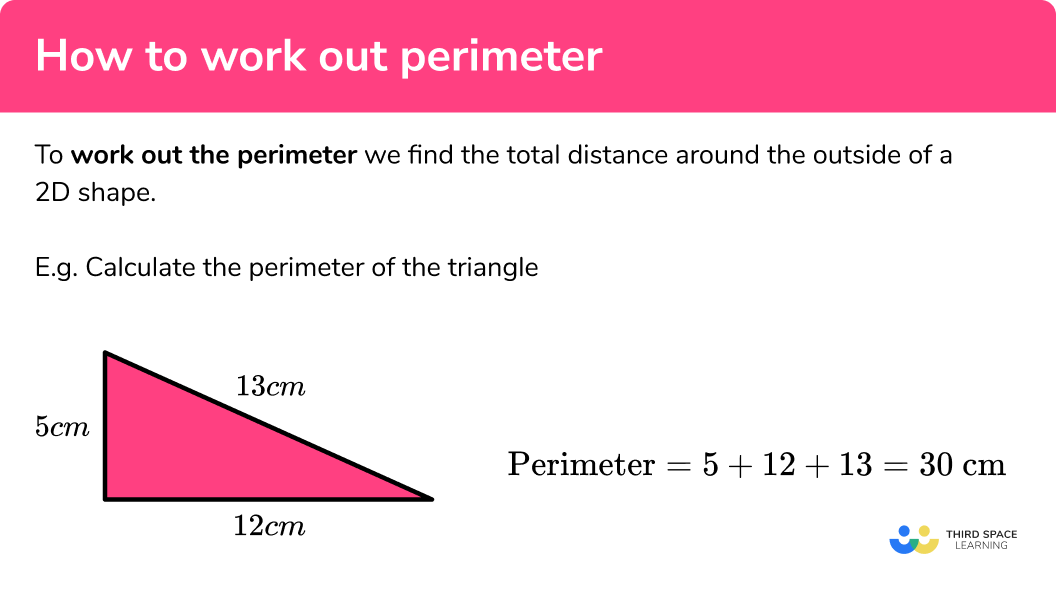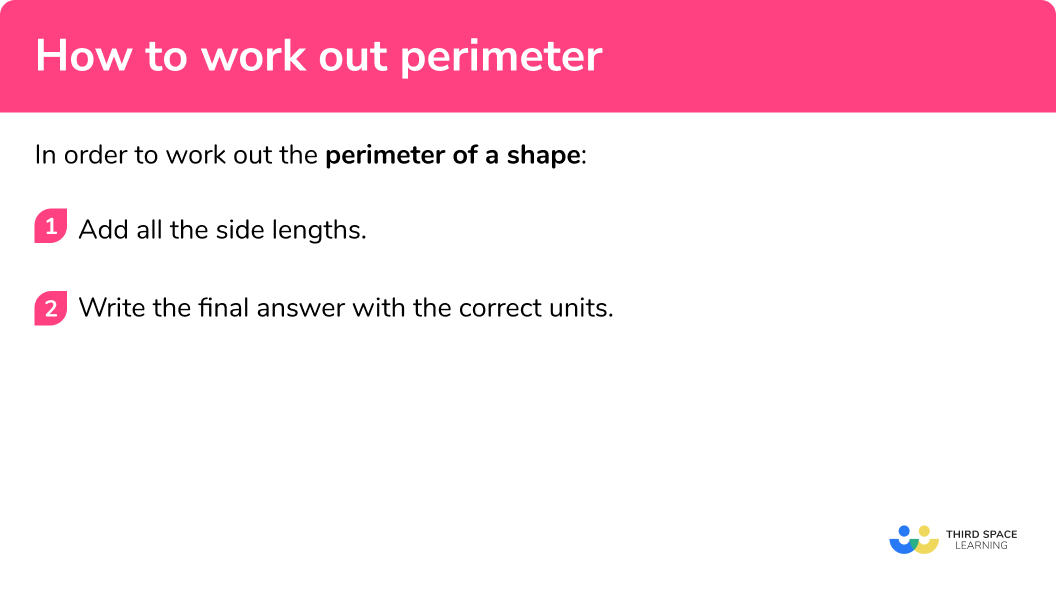GCSE Maths Geometry and Measure 2D Shapes

How To Work Out Perimeter

# How To Work Out Perimeter

Here we will learn how to work out perimeter, including what the perimeter of a shape is and how to calculate it for different shapes. We will also look at perimeter word problems.

There are also how to work out perimeter worksheets based on Edexcel, AQA and OCR exam questions, along with further guidance on where to go next if you’re still stuck.

## What is working out perimeter?

Working out perimeter is finding the total distance around the outside of a 2D shape.

For example,

Calculate the perimeter of the triangle.

\text{Perimeter}=5+12+13=30 \ \text{cm}

Step-by-step guide: Perimeter of compound shapes

### What is working out perimeter?### Perimeter of rectangles

The opposite sides of a rectangle are equal, a and b are the side lengths.

The perimeter of the rectangle is

\text{Perimeter}=a+b+a+b=2a+2b \ \text{or} \ 2(a+b)

### Perimeter of circles

The perimeter of a circle is known as the circumference of a circle.

\text{Circumference} = \pi \times d = 2 \times \pi \times r

Step-by-step guide: Circumference of a circle

## Perimeters of triangles and quadrilaterals

We can calculate the perimeter of any polygon (2D shape) by adding together the lengths of all the sides.

For example, calculate the perimeter of the triangle. All measurements are in cm.

\text{Perimeter}= 25 + 25 + 14 = 64 cm

Calculate the perimeter of the trapezium. All measurements are in cm.

\text{Perimeter} = 10 + 14 + 10 + 30 = 64 cm

For example, calculate the perimeter of the parallelogram. All measurements are in cm.

\text{Perimeter} = 8 + 4 + 8 + 4 = 24 cm

## How to work out the perimeter

In order to work out the perimeter of a shape:

1. Add all the side lengths.
2. Write the final answer with the correct units.

### How to work out the perimeter## How to work out perimeter examples

### Example 1: irregular shape

Find the perimeter of this triangle.

1. Add all the side lengths.

16+6+11=33

2Write the final answer with the correct units.

The correct units for this question are centimetres.

The perimeter is 33 \; cm

### Example 2: irregular shape

Find the perimeter of this quadrilateral.

Add the side lengths together. Make sure that you get each side.

8+5+6+5=24

The correct units for this question are centimetres.

The perimeter is 24 \; cm .

### Example 3: rectangle

Find the perimeter of this rectangle.

Add the side lengths together. Remember that the opposite sides of a rectangle are equal.

7+2+7+2=18

Alternatively, we can use a perimeter formula to find the perimeter of a rectangle.

\text{Perimeter}=2a+2b=2\times 7+2\times 2=14+4=18

OR

\text{Perimeter}=2(a+b)=2(7+2)=2\times 9=18

The correct units for this question are centimetres.

The perimeter is 18 \; cm .

### Example 4: rectangle

Find the perimeter of this rectangle.

Add the side lengths together. Remember that the opposite sides of a rectangle are equal.

5.1+9.4+5.1+9.4=29

Alternatively, we can use a perimeter formula to find the perimeter of a rectangle.

\text{Perimeter}=2a+2b=2\times 5.1+2\times 9.4=10.2+18.8=29

OR

\text{Perimeter}=2(a+b)=2(5.1+9.4)=2\times 14.5=29

The correct units for this question are centimetres.

The perimeter is 29 \; cm .

### Example 5: regular polygon

Find the perimeter of this square.

Add the side lengths together. Remember that all the sides of a regular shape such as a square are equal.

3.7+3.7+3.7+3.7=14.8

Alternatively, we can multiply the number of sides by the side length.

4\times 3.7=14.8

The correct units for this question are centimetres.

The perimeter is 14.8 \; cm .

### Example 6: regular polygon

Find the perimeter of this regular hexagon.

Add the side lengths together. Remember that all the sides of a regular shape such as a square are equal.

8.2+8.2+8.2+8.2+8.2+8.2=49.2

Alternatively, we can multiply the number of sides by the side length.

6\times 8.2=49.2

The correct units for this question are millimetres.

The perimeter is 49.2 \; mm .

## How to solve word problems involving perimeter

In order to solve word problems involving perimeter:

1. Consider the shape in the question and how to find its perimeter.
2. Work out the missing side.

### Example 7: word problems

A triangle has a perimeter of 18 \; cm.

Two of its sides are 7 \; cm.

Find the missing side.

The shape is a triangle.

To find the perimeter of a triangle we find the sum of the lengths.

We can work out the missing side by subtracting the two sides in the question from the perimeter.

18-(7+7)=18-14=4

The missing side is 4 \; cm .

### Example 8: word problems

A rectangle has a perimeter of 21 \; cm.

The shortest side is 4 \; cm.

Find the length of the longest side.

The shape is a rectangle.

The formula for finding the perimeter of a rectangle is,

\text{Perimeter}=2(a+b) .

We can work out the missing side by dividing the perimeter by 2 , and then subtracting the side length given in the question.

(21\div 2)-4=10.5-4=6.5

The longest side is 6.5 \; cm .

Alternatively you can rearrange the perimeter formula.

\begin{aligned} \text{Perimeter}&=2(a+b)\\\\ 2(a+4)&=21 \\\\ a+4&= 10.5\\\\ a&=6.5 \end{aligned}

### Common misconceptions

• Order of adding the sides

It doesn’t matter the order in which the sides of the shape are added.

• Check the units

Check which units are involved in the question as there may be a mixture.

### Practice how to work out perimeter questions

1. Find the perimeter of this triangle.19.2 \; cm19.3 \; cm19.1 \; cm19.4 \; cmAdding up all the side lengths gives

7.1+6.9+5.3=19.3 \ \text{cm} .

2. Find the perimeter of this rectangle.26.9 \; cm21.4 \; cm21.2 \; cm26.88 \; cmAdding up the short and long side and then doubling gives

2\times (6.4+4.2)=2\times 10.6=21.2 \ \text{cm} .

3. Find the perimeter of this regular polygon.36.4 \; mm36.6 \; mm36.7 \; mm36.5 \; mmFor a regular polygon we can multiply the length of one side by the number of sides,

5\times 7.3=36.5 \ \text{mm} .

4. The perimeter of this triangle is 21.6 \; cm . Find the missing side of this triangle.4.3 \; cm4.2 \; cm4.1 \; cm4.5 \; cmSubtracting the side lengths from the perimeter gives

21.6-(8.1+9.2)=21.6-17.3=4.3 \ \text{cm} .

5. The perimeter of a rectangle is 35.6 \; mm . The shorter side is 4.9 \; mm . Find the length of the longer side.

12.9 \; mm12.8 \; mm12.7 \; mm12.6 \; mmDividing the perimeter by 2 and then subtract the given side,

(35.6\div 2)-4.9=12.9 \ \text{mm} .

6. The perimeter of a regular octagon is 29.6 \; mm. Find the length of one of the sides of this regular octagon.

3.7 \; mm3.6 \; mm3.5 \; mm3.4 \; mmDividing the perimeter by the number of sides gives

29.6\div 8=3.7 \ \text{mm} .

### How to work out perimeter GCSE questions

1. The perimeter of a square is 36 \; cm.

What is the side length of the square?

(1 mark)

\text{B}-9 cm

(1)

2. A shape is formed from a rectangle measuring 11 \; cm by 14 \; cm from which a rectangle of length 7.1 \; cm has been removed.Find the perimeter of the shape.

(3 marks)

14-4.8-5.9=3.3

(1)

11+14+11+5.9+7.1+3.3+4.8

(1)

64.2

(1)

3. The diagram shows a regular hexagon and a rectangle.The perimeter of the regular hexagon is the same as the perimeter of the rectangle.

The length of the rectangle is 9 \; cm.

Work out the width of the rectangle.

(3 marks)

6\times 5=30

(1)

(30\div 2)-9

(1)

6

(1)

## Learning checklist

You have now learned how to:

• Work out the perimeter of irregular geometric shapes
• Work out the perimeter of a rectangle
• Work out the perimeter of a regular polygon
• Problem solve involving perimeter

## Still stuck?

Prepare your KS4 students for maths GCSEs success with Third Space Learning. Weekly online one to one GCSE maths revision lessons delivered by expert maths tutors.

Find out more about our GCSE maths tuition programme.